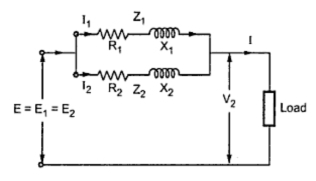### Parallel Operation of Transformers With Equal Voltage Ratios

Parallel Operation of Transformers With Equal Voltage Ratios
Let us now consider the case of two transformers connected in parallel having equal voltage ratios. The two transformers are having no load secondary voltage same. i.e. E1 = E2 = E. These voltages are in phase with each other. This is possible if the magnetizing currents of the two transformers are not much different. With this case the primaries and secondaries of the two transformers can be connected in parallel and no current will circulate under no load condition. This is represented in the Fig. 1.Fig. 1

If we neglect magnetizing components, the two transformers are represented as shown in the Fig. 2.Fig. 2

The phasor diagram under this case is shown in the Fig. 3. The two impedances Z1 and Z2 are in parallel. The values of Z1 and Z2 are with respect to secondary.Fig. 3

Z1 and Z2 are in parallel therefore the equivalent impedance is given by,
1/Zeq = 1/Z1 + 1/Z2            Zeq = Z1 Z2 /(Z1 + Z2 )
As seen from the phasor diagram
I1 Z1 = I2 Z2 = I Zeq
I1 = I Zeq/ Z1 = I Z2 /( Z1 + Z2 )
I2 = I Zeq/ = I Z1/( Z1 + Z2 )
Multiplying both terms of above equation by voltage V2,
V2 I1 = = V2 I Z2 /( Z1 + Z2 )
V2 I2 =  V2 I Z1/( Z1 + Z2)
But V2 I x 10-3 is Q i.e. the combined load in KVA
From this KVA carried by each transformer is calculated as,

The above expressions are useful in determining the values of QA and QB in magnitude and in phase.
Key point : The equation contains impedance ratio hence ohmic values of resistances and reactances are not required.
The two transformers work at different power factor. One operates at high p.f. while the other at low p.f. If the impedances Z1 and  Z2 are equal both in magnitude and quality i.e. ( X1/ R1 = X2/R2 ), both transformers operate at the same p.f. which is the p.f. of the load.
This can be solved graphically also. First we have to draw currents I1 and I2 with angular difference of (Φ1 - Φ2 ) and magnitude inversely proportional to the respective impedances with some suitable scale. The phase sum of I1 and I2 gives total current I. From loading condition the phase angle and magnitude of I can be obtained which can give the values of currents I1 and I2 can be determined.

1.2.